×
Get Full Access to UT - Study Guide - Final
Get Full Access to UT - Study Guide - Final

×

UT / Chemistry / CHEM 120 / Differentiate frequency from the wavelength.

# Differentiate frequency from the wavelength. Description

##### Description: This study guide covers everything that will be on Yang's final exam.
15 Pages 194 Views 3 Unlocks
Reviews

fhoward5 (Rating: )

CHEM 120 FINAL REVIEW

## What is dalton’s atomic theory?Chapter R:

Measurement – units and numbers

o significant figures (used in all calculations including the certain digits and the first  uncertain digit)

o rules for multiplication/division: same amount of SIGFIGS as the least precise  measurement

o rules for addition/subtraction: same number of decimal places as the least precise  measurement

o precision: agreement between several measurements

o accuracy: agreement of a value with the actual value  Don't forget about the age old question of The delta subunit that binds to calcium is called?

o random error: (intermediate error) measurement has an equal probability of being  too high or too low occurs when estimating the last digit of a measurement  o systematic error: (determinate error) occurs in the same direction every time always high or always low

## What is electron contrifugation?We also discuss several other topics like William hershel counted stars in how many regions?

o prefixes you need to know: kilo (10^3), deci (10^­1), centi (10^­2), milli (10^­3), micro  (10^­6)

o mass: a measure of the resistance of an object in order to change its state of motion NOT  TO BE CONFUSED WITH…

o weight: the force that is exerted on an object by gravity (used to measure mass) o EX) body mass does not change between the moon and earth, but weight does! o Density: the mass of a substance per unit volume (aka d=m/v) If you want to learn more check out What are the two critical differences between the halo and disk stars?

o Energy: the ability to do work or produce heat

o No energy can be created or destroyed (law of conservation of energy) o Kinetic energy: the energy of motion

o Potential energy: stored energy due to position

## It is a force that holds atoms together in a compound. what is it?o Dimensional analysis – (significant figures are required in all calculations) the process  that is taken in order to convert results from one unit to another

o Mole – concept, Avogadro’s number 6.022 x 10^23(Na), conversion between moles and numbers of particles (1 mole = 6.022 x 10^23 particles/molecules/atoms) o Classification of matter – matter is anything that occupies space and has mass o by state: gas (no fixed volume or shape, takes the shape of its container,  compressible), liquid (fixed volume and indefinite shape), solid (rigid, has fixed  volume and shape)

 by composition: pure substance (constant composition), element,

compound; mixture: variable composition homogeneous/solution  We also discuss several other topics like Who was orpheus?

(visibly indistinguishable parts) heterogeneous (having visibly

distinguishable parts)

o Separation of the mixtures: distillation (depends on differences in volatility), filtration  (solid and liquid are separated), chromatography (deals with a mobile phase and a  stationary phase)

Chapter 1:

o The law of conservation of mass: mass is neither created nor destroyed during a chemical Reaction (only transferred)

o The law of definite proportions: a given compound always has the same proportion of  elements by mass

o The law of multiple proportions: when 2 elements form a series of compounds, the ratios  of the masses of the 2nd element that combine with one gram of the first element can be  reduced to small whole numbers

o Dalton’s atomic theory: each element is made up of tiny particles called atoms, the atoms  of a given element are identical, chemical compounds are formed when atoms of different elements combine with each other

o Atomic structure

o Protons (positive charge equal to magnitude of an electron’s charge), electrons  (negative), neutrons (neutral and same mass as a proton)

∙ Isotopes: atoms with different numbers of neutrons We also discuss several other topics like L: v - v, what are the eigenvalues?

∙ atomic number(Z) number of protons, mass number (A) total  Don't forget about the age old question of How do black and white's teens differentially experience sibling and best friends' influence on substance abuse?

number of protons and neutrons

o Ions – cations (positively charged ions formed by losing electrons) and anions (negatively charged ions formed by gaining electrons)

Chapter 2:

o Electromagnetic radiation – radiant energy that exhibits wavelike behavior and travels  through space at the speed of light wave nature

o Wavelength: distance between two consecutive peaks/troughs (short

wavelength=high frequency and c =  ) λν

o Frequency: number of waves per second that passes a given point

o Classification of electromagnetic radiation:o Photoelectric effect: refers to phenomenon in which electrons are emitted from the surface of a  metal when light strikes it

o Light exhibits both wave and particle properties (there is a frequency threshold that must  be met in order for electrons to be emitted)

o energy of ONE photo: E = h  = hc/ ν λ

o Atomic spectra: line spectrum indicate that only certain energies are allowed for a particular  electron (aka electron energy levels are quantized)

o Bohr’s model for H atom: Bohr developed a quantum modelthe electron in a hydrogen atom  moves around the nucleus in circular orbits

o An atom loses energy when it jumps from higher n to lower n because the electron moves closer to the nucleus

o   Be able to explain and calculate atomic spectra of H atoms

o Quantum mechanical model – use wave function to describe electrons in atom; the  electron is assumed to behave as a standing wave

o wave function (Ψ): the function of the coordinates of the position of the electron  in 3D space (a specific wave function = orbital)

o probability distribution or probability density or electron density (Ψ2): the square  of the wave function/orbital indicates the probability of finding an electron near a  particular location

o atomic orbitals or orbital: a specific wave function for an electron

o four quantum numbers describe various parts of orbitals

 n (principal quantum #) 1, 2, 3, …; related to size and shape of orbital

 l (angular momentum quantum #) integrals from 0 to n­1; related to the  shape of the orbital (s (0), p (1), d (2), and f (3))

 ml (magnetic quantum #) integrals from ­l to +l; related to the orientation of the orbital

 ms electron spin (+1/2 or ­1/2); an orbital can only hold 2 electrons

o shell (refers to n), subshell (set of orbitals with a given angular momentum  quantum # aka values of l), orbital (n2)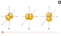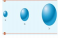o shapes of orbitals and representation of orbitals

 s orbital: sphere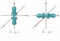 p orbital: peanut shape d orbital: four lobes

 f orbital: everything else

**IN A GIVEN ATOM, NO TWO ELECTRONS CAN HAVE THE SAME SET OF**  4 QUATUM NUMBERS (Pauli exclusion principle (also electrons have opposite spins))

o Polyelectronic atoms – an atom with more than one electron (similar shapes as orbitals  for H, but sizes and energies are different)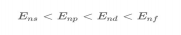o Periodic table –Mendeleev’s periodic table was organized so that elements with similar  properties were in the same vertical columns; the modern periodic table lists elements  based on atomic number

o Periods: rows

o groups (families): columns

 main group elements (A)

 transition & inner transition elements (B)

 metals (left): low ionization energies (easier to lose electrons), small

electron affinities (don’t like to add electrons), tend to form cations

 nonmetals (right): high ionization energies (harder to lose electrons), large negative electron affinities (like to add electrons), tend to form anions

 metalloids (along the line): elements exhibit both metallic and nonmetallic properties

 alkali metals (Li, K, Rb, Cs, and Fr): low ionization energies and chemical reactive; H has nonmetallic characteristics due to small size

o Electron configuration – description of orbitals containing electrons (1s22s22p63s2);  orbital diagrams are the representation of orbitals and half arrows that represent electrons o Rules

 Aufbau’s Principle: electrons occupy orbitals of low energy first (1s, 2s,  2p, 3s, 3p…)

 Ground state = electrons occupy the lowest energy level

 Excited state = electrons occupy other orbitals

 Pauli’s Exclusion Principle: one orbital can only hold two electrons with  opposite spins

 Hund’s Rule: electrons in the same subshell occupy orbitals one at a time  before they are paired

o Condensed electron configuration: take the noble gas from row above and  continue electron configuration

o valence electrons: for main group elements, electrons in high energy levels  (number of valence electrons = group number for main group elements) **Chromium and Copper have exceptions!!**

o core electrons: electrons in lower energy levels

o main group elements (s­block and p­block), transition elements (d­block), inner  transition elements (f­block)

Effective nuclear charge (Zeff) – net (positive) charge attracting electrons; increases from left to  right across a row, and does not change going down a group (more protons = stronger pull on  electrons)

o Atomic radii – the covalent atomic radius is one half of the distance between covalently  bonded nuclei; increases in going down a group (orbital size increases) and decreases  going from left to right (effective nuclear charge increases so valence electrons are pulled closer to nucleus)

o Ionization energy: the amount of energy required to remove an electron from the ground  state of a gaseous atom or ion (requires the input of energy to remove an electron); the

lower the IE, the easier it is to remove an electron and the more electrons removed, the  harder they become to be removed (IE1 < IE2 < IE3)

o The first ionization decreases in going down a group (electrons being removed are farther from the nucleus) and increases in going left to right across a row  (effective nuclear charge is greater)

­ Exception: Oxygen’s ionization is lower than Nitrogen’s.  Why? If you  look at the orbital notation, you will find that Nitrogen’s 2p orbitals are

half filled meaning it will be harder to remove those electrons

Electron affinity – the energy change associated with the addition of an electron to a gaseous atom or ion

o Becomes more negative when going from left to right (halogens have the most  negative electron affinities); no trend in groups

Chapter 3:

Chemical bonds – force that holds atoms together in a compound

Representing molecular compounds –

o chemical formula: shows the type and number of each element in a compound o empirical formula: shows relative number of each element in a compound o structural formula: shows the way atoms are bonded (think Lewis structure) ­ space filling model: indicates relative sizes of atoms as well as their

relative orientation in the molecule

­ ball and stick method: 3D model using spheres and rods

Octet rule: a chemical bond is the sharing or transfer of electrons to attain a stable electron  configuration for the bonding atoms; elements seek to obtain noble gas electron configuration

Ionic bonds and ionic compounds: transfer of electrons from a metal to a nonmetal; electrostatic  attractive force between oppositely charged ions

o Predicting charges of ions and formulas of ionic compounds

­ For most main group elements, the charge of cation = group number and  the charge of anion = group number ­8

Keep in mind ions are charged particles, but ionic compounds are electrically neutral! o Electron configuration of ions

o Sizes of ions: influences structure and stability of ionic solids and it is determined by measuring the distance between ion centers

­ The size of the parent ion and its position in the periodic table influences  the size of an ion

­ Cations are smaller than their parents, while anions are larger than their  parents

o isoelectronic ions: ions with the same number of electrons

­ size decreases with an increasing effective nuclear charge

o Lattice energy: energy that takes place when separated gaseous ions are packed  together to form an ionic solid

­ k (Q1*Q2 / r)

­ compounds with smaller ions and higher charges have higher lattice

energies

Covalent bonds and molecular compounds: the sharing of electrons between two atoms  (interaction between nonmetals); covalently bonded atoms compose a molecule = molecular  compounds; ionic compounds form when cations and anions are bonded together by ionic bonds

o Localized electron (LE) bonding model (pairs of electrons on an atom = lone pairs and electron pairs found in the space between atoms = bonding pairs)

­ Description of valence electron arrangement in the molecule using Lewis  structures

­ Prediction of geometry of the molecule using valence shell electron­ pair  repulsion model

­ Description of the type of atomic orbitals used by the atoms to share

electrons or hold lone pairs

o Polar covalent bond: electrons are unequally shared

o Nonpolar covalent bond: electrons are equally shared

Arrow points to the more electronegative atom (­)

o Electronegativity – the ability of an atom in a molecule to attract bonding  electrons to itself

­ Increases across a period and decreases down a group (fluorine is the most electronegative element)

­ use electronegativity difference of two atoms to predict bond polarity; the  greater the difference in electronegativity, the more polar the bond

o Lewis structures – show how valence electrons are arranged among the atoms in a molecule  atoms achieve noble gas electron configuration (octet rule)

­ Write the correct skeletal structure for the molecule (central atom is the  least electronegative atom)

­ Determine the total number of valence electrons each atom is bringing in  to form the molecule

­ Distribute the electrons among the atoms in the molecule giving octets to  as many as possible

­ If any atom lacks an octet, form double/ triple bonds as necessary

Remember multiple bonds are shorter and stronger than a single bond thus has a greater bond energy!

­ Resonance: when a molecule has multiple structures to describe the

molecule  the resulting structure is derived from the average of the

resonance structures

­ Formal charge (helps us choose between alternative Lewis structures fewest charges and negative charge put on the most electronegative atom

= better Lewis structure): occurs when atoms end up with more of fewer

electrons than the valence electrons they brought in order to fill their

octets

o (Valence electrons) – (nonbonding electrons) – (1/2 bonding

electrons)

­ Exceptions to octet rule: odd­electron species, incomplete octets, and  expanded octets

o Odd­electron species: molecules/ions with odd number electrons =

o Incomplete octets: Some molecules have less than an octet of

electrons (Be, B, and Al)

o Expanded octets: central atom has more than eight electrons

around it (occurs in 3rd row or below elements)

o   Bond length: distance between nuclei of bonded atoms (triple bond < double bond < single bond)

o Bond energy: amount of energy needed to break a bond (triple bond > double  bond > single bond)

Naming ionic and molecular compounds –

o Type 1: cation is named first, and anion is second (cation is name of element and  anion is root of the element plus “ide”)

o Type 2: some metals form more than one type of positive ion (charge is specified  by roman numeral)

­ Alternatively, for metals that form only two ions, ions with higher charge  end in “­ic”, and ions with lower charge end in “­ous”

o Type 3: formed between two non­metals

­ first element is named, then the second is named as if it were an anion ­ prefixes are used to indicate the number of atoms present

Polyatomic Ions­ consist of atoms joined as in a molecule, but they have charge

KNOW THESE:

Acetate: C2H3O2­

Carbonate: CO32­

Hydroxide: OH

Nitrate: NO3­

Phosphate: PO43­

Ammonium: NH4+

Sulfate: SO42­

Perchlorate: ClO4­

Chapter 4 (sections 1­3):

Valence Shell Electrons Pair Repulsion Model (VSEPR): the structure around a given atom is  determined principally by minimizing electron repair repulsions

o electron geometry: arrangement of electron pairs (lone pairs are physically larger  than bonding electron pairs thus more repulsion leads to smaller bond angles) o molecular geometry: arrangement of atoms

when there are no lone pairs, electron geometry = molecular geometry, but when there are lone pairs, electrons geometry does not equal molecular geometry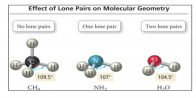Bond polarity: covalent bond formed between two atoms with different electronegativities are  polar; a molecule that has a center positive charge and a center of negative charge is said to have  a dipole moment

1. predict Lewis structure

2. use VSEPR theory to predict molecular shape

3. use electronegativity values to predict bond dipoles

4. determine polarity of a molecule based on overall dipole moment of molecule

Hybridization: procedure of mixing the native atomic orbitals to form special orbitals for  bonding

o a new set of degenerate orbitals formed from hybridization = hybrid orbitals o sp3 hybridization: tetrahedral arrangement with 109.5° bond angles

o sp2 hybridization: trigonal planar arrangement with 120° bond angles  o sp hybridization: linear geometry with 180° bond angles

o sp3d hybridization: trigonal bipyramidal geometry with 120° and 90° bond angles o sp3d2 hybridization: octahedral geometry with 90° bond angles

Molecular orbital (MO) theory

bonding orbitals: lower in energy than the atomic orbitals of which it is composed

antibonding orbitals: higher in energy than the atomic orbitals of which it is composed molecular orbital diagram – be able to draw MO diagram formed from s atomic orbitals          MO diagram from p atomic orbitals will be given.

*to participate in molecular orbitals, atomic orbitals must overlap in space*

bond order: the difference between the number of bonding electrons and number of  antibonding electrons divided by 2; it is an indication of bond strength  larger bond  order = greater bond strength and the shorter the bond length

predict stability of molecules or ions: molecule is more stable when it has a lower energy  than its separated parts; if bonding it favored, the molecule will be stable and form a  bond

paramagnetic: causes the substance to be attracted into the inducing magnetic field  leads to increase in weight when magnetic field is turned on  UNPAIRED  ELECTRONS

diamagnetic: causes the substance to be repelled from the including magnetic field  PAIRED ELECTRONS

Chapter 5:

Basics

calculating atomic mass from isotopes: mass abundance multiplied by the atomic mass  units  then add them together

mass spectrum: graph of mass of an element (isotope) versus the relative number of  atoms (relative abundance)

formula mass: sum of the atomic masses for the atoms in a chemical formula  used to  calculate molar mass in compounds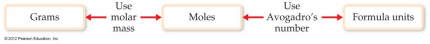Stoichiometry based on chemical formulas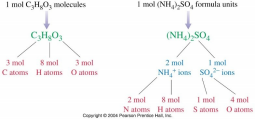­ Percent composition of compounds: mass of element in one mole of compound / mass of one mole of compound times 100%

­ Determining empirical formulas: obtain the mass of each element and convert to  moles, divide all by the smallest number of moles

­ Determining molecular formulas: empirical formula multiplied by n n = molar  mass / empirical formula molar mass

Stoichiometry based on chemical equations

­ Balancing chemical equations: always start with the most difficult compound first,  and leave stand­alone elements for last; in combustion reactions (O2 is a reactant and  Co2 and H2O are products), start with C, then H, then O; think conservation of  mass

­ Information from balanced chemical equations they tell us the physical states of  compounds/ molecules, coefficients tell us number of molecules, and subscripts tell  us number of atoms in a molecule

­ Stoichiometry calculations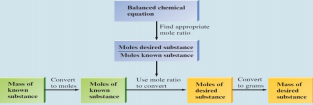­ Limiting reactant: the reactant that limits the amount of product forming; makes the  least amount of product

­ Excess reactant: the reactant that is left over; not all of it is used in the chemical  reaction

­ Theoretical yield: the amount of product that can be made based on the among of  limiting reactant

­ Percent yield: actual yield / theoretical yield x 100%

Chapter 6:

Solution formation

Conductivities of aqueous solutions – strong electrolytes fully ionize (acids – HCl, HBr, HI,  H2SO4, HNO3, HClO4 and bases­ group 1 A metal hydroxides and heavy metal group 2A  hydroxides), weak electrolytes partially ionize (reaction can go in either direction),  nonelectrolytes (do not conduct electricity and do not produce ions)

Molarity: M = n/V

Dilution: M1V1 = M2V2 *(ONLY FOR DILUTIONS)*

Precipitation reactions  only occur when a precipiation forms

­ predicting if a salt is soluble in water (most salts are soluble) or not using solubility  rules

­ formula equation: balanced equation

­ complete ionic equation: all strong electrolytes dissociate into ions

­ net ionic equation: show the species acutally involved (spectator ions are the ions that are cancelled out or didn’t change from the reaction)

Acid­base reactions

­ acid: substances that ionize in water form H+

­ base: substances that ionize in water from OH

strong electroylytes completely ionize in water and weak electrolytes partially ionize in water

­ acid­base reation / neutralization reaction: an acid and a base react together and they  neutralize each other, producing water and salt net ionic equations end up being H+  + OH­  H2O

­ titration:  technique used to calculate the concentration of a solute (equivalence points is when moles of H+ = OH

Oxidation­reduction reactions

­ oxidation state (or oxidation number): number assigned to each element in a reaction  that determines the electron flow in the reaction

­ oxidation­reduction / redox reaction: electrons are transferred from one reactant to the other (“OIL RIG”  Oxidation Is Losing, Reduction Is Gaining)

­ oxidizing agent: reactant that is reduced and oxidizes other substance

­ reducing agent: reactant is oxidized and reduces other substance

­ balancing oxidation­reduction equations: assign oxidation states and use tie lines to  show electrons gained and lost (make them equal by multiplication).  DON’T

FORGET TO BALANCE THE REST OF THE ELEMENTS

Solution stoichiometry

StoichiometricM = n/V coefficients M = n/VChapter 7: (sections 1­5)

o The first law of thermodynamics – energy is neither created nor destroyed (only transferred) and  total energy of the universe is CONSTANT; delta E = internal energy (sum of kinetic and  potential energies of all the particles in the system) ΔE = E final – E initial

o internal energy of a system can be exchanged within the surroundings through heat and  work

 state function: property of the system that depends only on its present state (not its path)

*ΔE = q + w*

 (+q)  system gains thermal energy; (­q) system loses thermal energy

 (+w)work done on the system; (­w)work done by the system

 (+ΔE) energy flows into the system, endothermic; (­ΔE) energy flows out of  the system, exothermic

o Heat: the exchange of thermal energy between the system and surroundings (heat  exchange ONLY occurs when there is a temperature difference); heat flows from high T  to low T until thermal equilibrium is achieved

o Heat capacity: amount of heat required to raise the temperature of a substance by 1  degree Celsius

*q = Cs*m*ΔT*

o Pressure­Volume Work: caused by a volume change against an external pressure (when gases expand, ΔV is positive but the system is doing work on the surroundings, so W is negative)

*w = ­ PΔV*

o Enthalpy, H – the total heat content of a system; at constant pressure, the change in enthalpy is  the heat gained or lost

*H = E + PV*

o Three properties of enthalpy

 It’s a state function (ΔH = H final – H initial)

 ΔH = qp (ΔH<0  exothermic, ΔH>0  endothermic)

o It’s an extensive property (depends on the AMOUNT of substance) CONSTANT  PRESSURE

o endothermic reaction: heat is absorbed, heat flows into the system, reactants have less  energy than the products

o exothermic reaction: heat is released, heat flows out of the system, reactants have more  energy than the products

o Enthalpy of reaction, ΔHrxn: depends on the states of the products and reactants (ΔH = H products –  H reactants

o Calorimetry: use a calorimeter (measures heat associated with chemical reactions)  ΔH = qpconstant pressure calorimetry is used to measure ΔH of a reaction, and  the heat produced is absorbed by solution; constant volume calorimetry is used to  measure ΔE (q cal = C cal *ΔT)

o Hess’s law: in going from a particular set of reactants to a particular set of products, the  change in enthalpy is same whether the reaction takes place in one step or a series of  steps

 ΔH rxn Holds the same properties of enthalpy

 If the chemical equation is multiplied by a factor, so is ΔH rxn

 If chemical equation is reversed, so is ΔH rxn

o Standard enthalpy change (ΔHo):  enthalpy change when all reactants and products are in  standard states

 Standard state: pure gas at 1atm, pure solid or liquid in its most stable form at 1  atm and 25 degrees Celsius, substances in solution with 1M concentration

 Standard state for elements is 0!!!!!!; graphite is the most stable state for carbon o Standard enthalpies of formation (ΔHfo) standard enthalpy change when one mole of  compound forms from its constituent elements in their standard state

 formation reactions: elements (standard state)  compound (standard state); ΔHfo   compound = ΔHo

 calculating ΔHrxno from ΔHfo: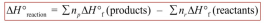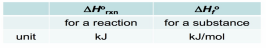Chapter 8: (sections 1­7)

o Gas: uniformilly fills a container, eaily compressible, mixes COMPLETELY with  another gas, and exerts pressure on its surroundings

o Gas Pressure: Force / Area (unit  N/m2 or Pa)

o Atmospheric pressure: weight of air per unit of area (standard = sea level), use a  barometer to measure atmospheric pressure (1atm = 760 mmHg = 760 torr) o Gas laws: equations expressing the relationship among T, P, V, and n o Boyle’s Law: P­V P1V1 = P2V2 (at constnat n and T, V is inversley proportional  to P)

o Charles’s Law: V­T  V1/T1 = V2/T2 (at constnat n and P, V is directly  proportional to absolute T which is 0K)

o Avagadro’s Law: V­n  V1/n1 = V2/n2 (at constant P and T, V is directly  proportional to the number of gas molecules)

 At constant T and P, when V is doubled, the number of moles is also  doubled

o Ideal Gas Law: V is propotional to nT/P or V = R * nT/P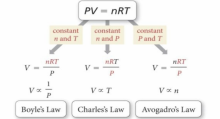*PV = nRT*

o UNITS ARE IMPORTANT!! Pressure in atm, Volume in liters, n in moles,  Temperautre in kelvin, and R = 0.0821 (L*atm)/(K*mol)

o   Applications of Ideal Gas Law

 In order to double the pressure of a gas, double the amout of gas, double  the Temperaure (in Kelvin), or decrease the volume by half

 When you increase the amount of gas by a factor of 3 and at the same time you increase the volume by a factor of 3, the pressure will remain

constant!

 If you increase the pressure by a factor of 3 and at the same time you  increase the temperautre by a factor or 3, the pressure will increase by a  factor of 9

 If you undersand how to derive equations from ideal gas law and

relatioships, then you will be able to apply the idela gas law

o STP

o Molar Volume: for 1 mole of ideal gas at 1 atm and 273.2 K  22.42L

o Gas stoichiometry

o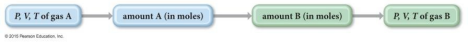Molar mass and density of gas: molar mass = mass (m)/ mole (n), so n = m/ molar mass *Molar mass= dRT/ P d = P*Molar Mass / RT*

o The higher the molar mass and pressure, the denser the gas!

o The higher the temperature, the less dense the gas

o At constant T and P, the higher the molar mass, the denser the gas

o Mixtures of gases

o Dalton’s law of partial pressures: for a mixture of gases in a container, the total pressure  exerted equals the sum of the pressures that each gas would exert if it were alone (P total =  P1+ P2 + P3 + …

 partial pressure: P1, P2, P3 are partial pressures where P1 = n1RT/V and so on  vapor pressure: partial pressure of water vapor depends on the temperature in  table (Ptotal = Pgas + Pwater)

o mole fraction: moles of one component in a mixture divided by the total moles (X1 =  n1/ntotal; ratio of partial pressure of a single gas and total pressure is the mole  fraction

Page ExpiredIt looks like your free minutes have expired! Lucky for you we have all the content you need, just sign up here